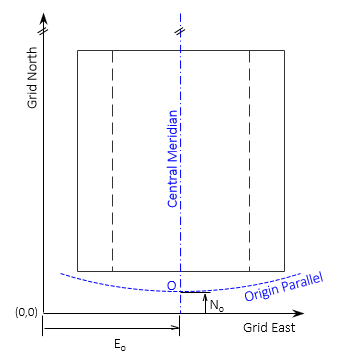### Article Index

A grid coordinate system is developed based on the needs of its users. It there are a spectrum of applications, the system must support the highest accuracy needs. Design criteria can be divided into two general categories: Coverage area and Projection parameters.

### 1. Coverage Area

#### a. Projection Type(s)

The shape of the area will determine if a cylindric or conic projection fits best. Consider designing statewide systems, Figure E-1:

• A conic projection would be appropriate for Tennessee because it is long east to west
• A cylindric projection would be for Alabama because it is long north to southFigure E-1 Selecting Appropriate Projections

A squarish state (geographically, not mentally), like Wyoming, could use either.

#### b. Maximum Distortion

The larger the area covered, the greater the distortions. Although distortions are systematic, a maximum is generally specified that is within acceptable limits for most users. This in turn will affect the amount of area that can be covered by a projection or even the type of projection used.

For example, a single cylindric projection for Alabama might not meet a specific maximum distance distortion. If the state is divided into north and south zones, a conic projection could be used for for each, Figure E-2.Figure E-2 Using Two Projections

Most regional coordinate systems are designed to meet a maximum distortion level. In the original State Plane Coordinate (SPC) systems, the limit was 1/10,000. As a result, most state SPC systems used multiple over-lapping zones since a single zone, like the Alabama example, allowed too much distortion.

#### (1) Zone Extent

Secant projections are preferred for creating regional coordinate systems because they allow scale to range from less than 1 to greater than 1. This balances the overall scaling effect.

Zone extent (ZE) is the maximum distance a zone extends in the direction of varying scale; north-south for a Lambert Conic, east-west for a Transverse Mercator Cylindric. The projection is fit so two-thirds of the ZE is between the scale = 1 lines, the remaining third is split into two equal distances outside. Figures E-3 and E-4 show how a conic and transverse cylindric projections are fit.Figure E-3 Conic Projection Fit

A transverse cylindric projection is fit similarly, Figure E-4, to achieve an averaging scale effect.Figure E-4 Transverse Cylindric Projection Fit

#### (2) Central Meridian

The Central Meridian (CM) defines Grid North for the coordinate system and its longitude locates the projection east-west.

### 2. Projection Parameters

Projection parameters define and locate the grid coordinate axes to the projection.

#### a. Lambert Conformal Conic, Figure E-5Figure E-5 Lambert Conic Projection Parameters

Along with the CM longitude, parameters for a conic projection define:

Latitudes of the North and South Standard Parallels (where scale = 1)
Latitude of the Grid Origin, O
False Northing and Easting which fix the axes origin, (0,0), to the Grid Origin.

#### b. Transverse Mercator Conformal Cylindric, Figure E-6Figure E-6 Transverse Mercator Cylindric Projection Parameters

Along with the CM longitude, parameters for a cylindric projection define:

Scale along the CM
Latitude and longitude of the Grid Origin, O
False Northing and Easting which fix the axes origin, (0,0), to the Grid Origin.

### 3. Coordinate Conversion

Because of the math connection between the ellipsoid and grid, coordinates on one can be converted to coordinates on the other, Figure E-7.Figure E-7Coordinate Conversion

Coordinate conversions are:

• Direct (or Forward) - geodetic to grid
• Inverse - grid to geodetic

Using the projection equations and parameters, measurable conversion errors will not be introduced.

### 4. Almost there...

We have most of the pieces, we just have to start putting things together.